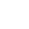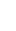HomeModulesArticlesVideosLife EventsCalculatorsQuizJargonExtras

### Investments

 + Investments Overview + Returns from investment + Asset classes - Asset class - cash + Asset class - fixed interest + Asset class - property + Asset class - shares + Tax and investment returns + Diversification and portfolio construction + Relationship between risk and return + Investment risk profiles + Investment methods + Analysis of performance + Performance of managed funds + Performance of investment portfolios + Summary of investments## Compound interest

If the interest was paid on a monthly basis and retained in the account the interest earned would differ from the previous example, in effect you would then be earning interest on interest – this is referred to as compound interest.

Using the same figures as the example above, if the interest was paid monthly, the monthly interest earned would 2.7% divided by 12, or 0.225%.

Therefore the interest paid in the first month would be:

\$1,000 x 0.225 / 100 = \$2.25 per month

This brings your bank balance to \$1,002.25 after the first month. For the second month, the interest will be calculated on your original \$1,000 plus the previous month’s interest of \$2.25. So you are earning interest on your interest.

We will show you an example of this on the next page.